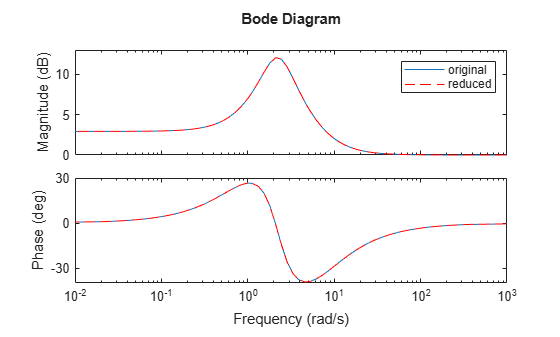Documentation

# isproper

Determine if dynamic system model is proper

## Syntax

```B = isproper(sys) B = isproper(sys,'elem') [B,sysr] = isproper(sys) ```

## Description

`B = isproper(sys)` returns a logical value of `1` (`true`) if the dynamic system model `sys` is proper and a logical value of `0` (`false`) otherwise.

A proper model has relative degree ≤ 0 and is causal. SISO transfer functions and zero-pole-gain models are proper if the degree of their numerator is less than or equal to the degree of their denominator (in other words, if they have at least as many poles as zeroes). MIMO transfer functions are proper if all their SISO entries are proper. Regular state-space models (state-space models having no `E` matrix) are always proper. A descriptor state-space model that has an invertible `E` matrix is always proper. A descriptor state-space model having a singular (non-invertible) `E` matrix is proper if the model has at least as many poles as zeroes.

If `sys` is a model array, then `B` is `1` if all models in the array are proper.

`B = isproper(sys,'elem')` checks each model in a model array `sys` and returns a logical array of the same size as `sys`. The logical array indicates which models in `sys` are proper.

`[B,sysr] = isproper(sys)` also returns an equivalent model `sysr` with fewer states (reduced order) and a non-singular `E` matrix, if `sys` is a proper descriptor state-space model with a non-invertible `E` matrix. If `sys` is not proper, `sysr = sys`.

## Examples

collapse all

Create a SISO continuous-time transfer function, ${H}_{1}=s$

`H1 = tf([1 0],1);`

Check whether `H1` is proper.

`B1 = isproper(H1)`
```B1 = logical 0 ```

SISO transfer functions are proper if the degree of their numerator is less than or equal to the degree of their denominator That is, if the transfer function has at least as many poles as zeroes. Since `H1` has one zero and no poles, the `isproper` command returns `0`.

Now create a transfer function with one pole and one zero, ${H}_{2}=s/\left(s+1\right)$

`H2 = tf([1 0],[1 1]);`

Check whether `H2` is proper.

`B2 = isproper(H2)`
```B2 = logical 1 ```

Since `H2` has equal number of poles and zeros, `isproper` returns `1`.

Combining state-space models sometimes yields results that include more states than necessary. Use `isproper` to compute an equivalent lower-order model.

```H1 = ss(tf([1 1],[1 2 5])); H2 = ss(tf([1 7],)); H = H1*H2; size(H)```
```State-space model with 1 outputs, 1 inputs, and 4 states. ```

`H` is proper and reducible. `isproper` returns the reduced model.

```[isprop,Hr] = isproper(H); size(Hr)```
```State-space model with 1 outputs, 1 inputs, and 2 states. ```

`H` and `Hr` are equivalent, as a Bode plot demonstrates.

```bodeplot(H,Hr,'r--') legend('original','reduced')```